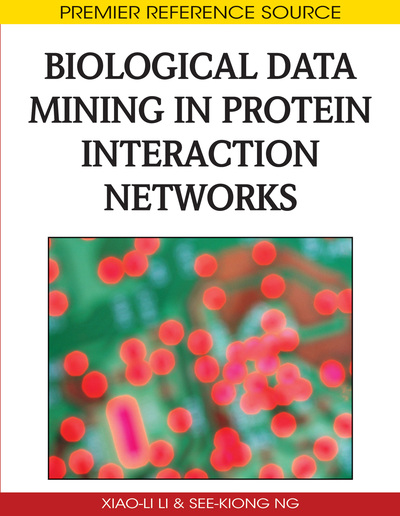# Domain-Based Prediction and Analysis of Protein-Protein Interactions

Tatsuya Akutsu (Kyoto University, Japan) and Morihiro Hayashida (Kyoto University, Japan)
DOI: 10.4018/978-1-60566-398-2.ch003

## Abstract

Many methods have been proposed for inference of protein-protein interactions from protein sequence data. This chapter focuses on methods based on domain-domain interactions, where a domain is defined as a region within a protein that either performs a specific function or constitutes a stable structural unit. In these methods, the probabilities of domain-domain interactions are inferred from known protein-protein interaction data and protein domain data, and then prediction of interactions is performed based on these probabilities and contents of domains of given proteins. This chapter overviews several fundamental methods, which include association method, expectation maximization-based method, support vector machine-based method, and linear programmingbased method. This chapter also reviews a simple evolutionary model of protein domains, which yields a scalefree distribution of protein domains. By combining with a domain-based protein interaction model, a scale-free distribution of protein-protein interaction networks is also derived.
Chapter Preview
Top

## Introduction

Understanding of functions of genes and proteins is important in post-genomic era. Information on protein-protein interactions is useful for understanding protein functions because protein-protein interactions play a key role in many cellular processes. Since the end of the last century, some experimental techniques have been developed for comprehensive analysis of protein-protein interactions, which include two-hybrid systems and proteomics methods. Though these experimental methods revealed many unknown interactions, there were large gaps between results done by different groups (Ito et al., 2001; Uetz et al., 2000). Therefore, computational methods should be developed for inference of protein-protein interactions. For that purpose, various approaches have been proposed. Since other approaches and aspects will be covered in other chapters in this book, this chapter focuses on computational and mathematical aspects of domain-based approaches.

A protein consists of one or multiple domains, where a domain is defined as a region within a protein that either performs a specific function or constitutes a stable structural unit. Examples of structural domains are illustrated in Fig. 1 though domains are sometimes defined not based on structures but based on sequence/functional similarities. In a word, domains are considered as parts of a protein. Though there is no exact or mathematical definition of protein domains, several hundreds of protein domains are currently known. In order to classify domains, several database systems have been constructed, which include Pfam (Finn et al., 2005), InterPro (Nicola et al., 2007) and ProDOM (Bru et al., 2005). Furthermore, most of these databases provide facilities to identify protein domains from a given protein sequence. In Pfam, each domain is represented by an HMM (Hidden-Markov Model) and protein domains contained in a given protein sequence are identified by using these HMMs.

Figure 1.

Example of protein domains. Protein P1 consists of domains D1 and D2, whereas protein P2 consists of domains D3, D4 and D5. In domain-based models, it is assumed that P1 and P2 interact with each other if at least one domain pair interacts

Utilizing information of domain organizations of proteins, several methods have been proposed for prediction of protein-protein interactions. In these methods, scores or probabilities of domain-domain interactions are first derived from known protein-protein interactions and then these are utilized for calculating the score or probability of protein-protein interaction for given protein sequences. Sprinzak and Margalit (2001) proposed the association method for computing the score of each domain pair. Kim et al. (2002) proposed similar scores and applied the scores to inference of protein-protein interactions. Deng et al. (2002) proposed an EM (Expectation-Maximization) algorithm for estimating the probability of interaction for each domain pair.

In these methods, it is assumed that protein-protein interaction data are given as binary data (i.e., whether or not each protein pair interacts is given). However, multiple experiments are performed for the same protein pairs in practice and thus the ratio of the number of observed interactions to the number of experiments is available for each protein pair. For example, Ito et al. (2001) performed multiple experiments for each protein pair. But, the results are not always the same. Therefore, it is reasonable to use the ratio of the number of observed interactions to the number of experiments as input data. We developed a method utilizing these ratios (Hayashida et al., 2003; Hayashida et al., 2004), which was further improved by Chen et al. (2006).

## Complete Chapter List

Search this Book:
Reset